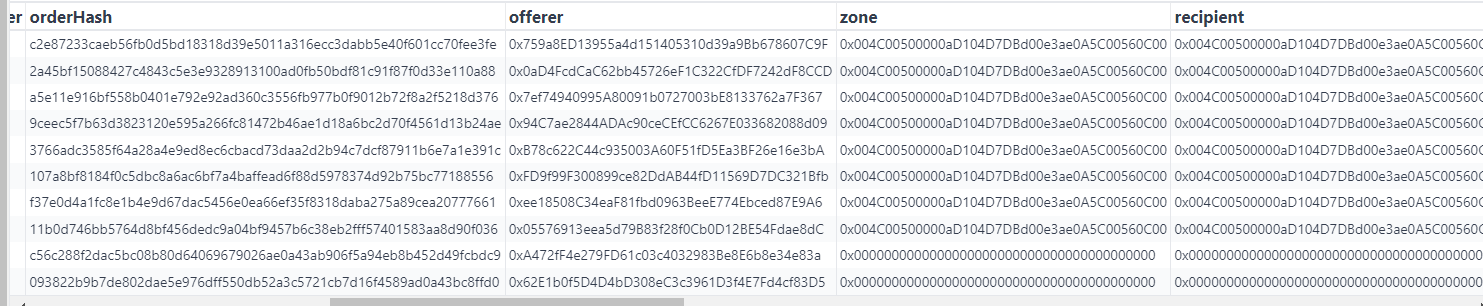## Get ERC20 Balance for an account

Examples for account `0x3ebd637f0fc8f1bac02cbd138916a8e2d7f5898a`

### Get all ERC20 token balances

``````WITH txfers AS (
SELECT
block_number,
transaction_hash,
symbol,
CASE WHEN from_address = '0x3ebd637f0fc8f1bac02cbd138916a8e2d7f5898a' THEN -cast(value as decimal)
ELSE cast(value as decimal)
END as value,
decimals,
END as peer,
row_number() OVER(PARTITION BY contract_address ORDER BY block_number DESC) AS row_number,
block_time,
data_creation_date
FROM
ethereum_mainnet.erc20_evt_transfer
WHERE 1=1
AND

-- uncomment to filter results on the contract address of the token
ORDER BY block_number DESC
)
SELECT
symbol,
decimals,
SUM(value / pow(10, decimals)) AS balance,
COUNT(1) AS num_of_txfers,
MIN(block_time) AS first_transfer_timestamp,
MAX(block_time) AS last_transfer_timestamp
FROM txfers
-- uncomment to get historical balance at a certain point of time
-- WHERE data_creation_date <= DATE('2022-09-01') AND block_time <= CAST('2022-09-01 06:00' AS timestamp)
LIMIT
200
``````

Output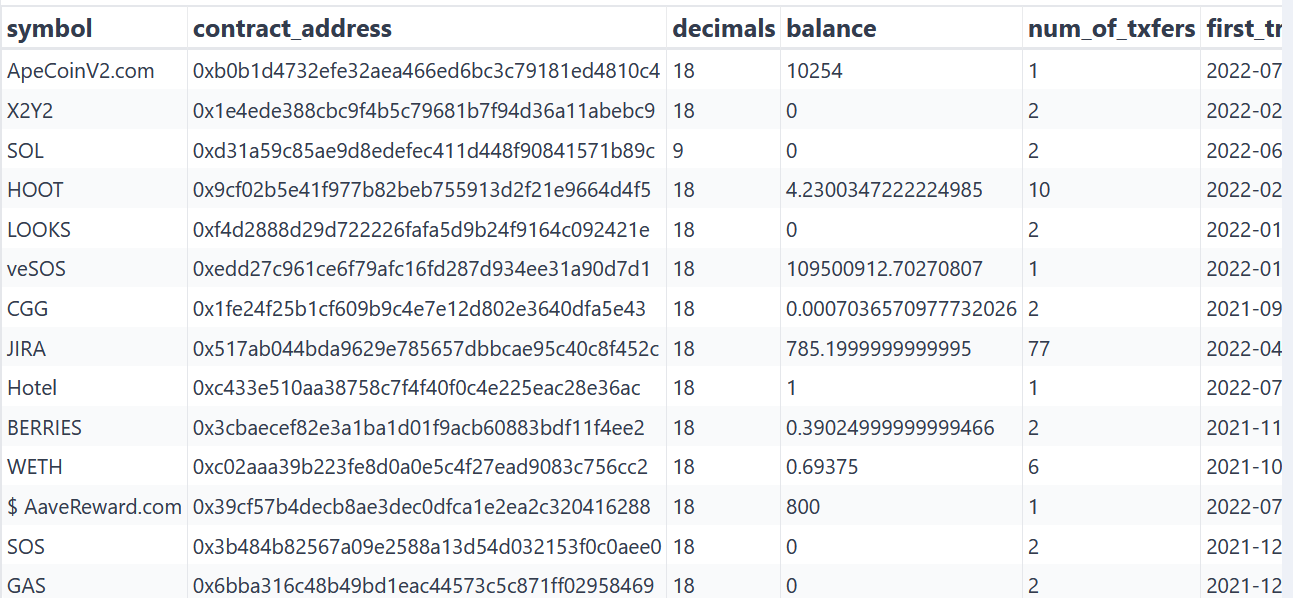### Filter results on the ERC20 token

``````WITH txfers AS (
SELECT
block_number,
transaction_hash,
symbol,
CASE WHEN from_address = '0x3ebd637f0fc8f1bac02cbd138916a8e2d7f5898a' THEN -cast(value as decimal)
ELSE cast(value as decimal)
END as value,
decimals,
END as peer,
row_number() OVER(PARTITION BY contract_address ORDER BY block_number DESC) AS row_number,
block_time,
data_creation_date
FROM
ethereum_mainnet.erc20_evt_transfer
WHERE 1=1
AND
ORDER BY block_number DESC
)
SELECT
symbol,
decimals,
SUM(value / pow(10, decimals)) AS balance,
COUNT(1) AS num_of_txfers,
MIN(block_time) AS first_transfer_timestamp,
MAX(block_time) AS last_transfer_timestamp
FROM txfers
-- uncomment to get historical balance at a certain point of time
-- WHERE data_creation_date <= DATE('2022-09-01') AND block_time <= CAST('2022-09-01 06:00' AS timestamp)
LIMIT
200
``````

### Get historical balances

``````WITH txfers AS (
SELECT
block_number,
transaction_hash,
symbol,
CASE WHEN from_address = '0x3ebd637f0fc8f1bac02cbd138916a8e2d7f5898a' THEN -cast(value as decimal)
ELSE cast(value as decimal)
END as value,
decimals,
END as peer,
row_number() OVER(PARTITION BY contract_address ORDER BY block_number DESC) AS row_number,
block_time,
data_creation_date
FROM
ethereum_mainnet.erc20_evt_transfer
WHERE 1=1
AND
-- uncomment to filter results on the contract address of the token
ORDER BY block_number DESC
)
SELECT
symbol,
decimals,
SUM(value / pow(10, decimals)) AS balance,
COUNT(1) AS num_of_txfers,
MIN(block_time) AS first_transfer_timestamp,
MAX(block_time) AS last_transfer_timestamp
FROM txfers
WHERE data_creation_date <= DATE('2022-09-01') AND block_time <= CAST('2022-09-01 06:00' AS timestamp)
LIMIT
200
``````

Output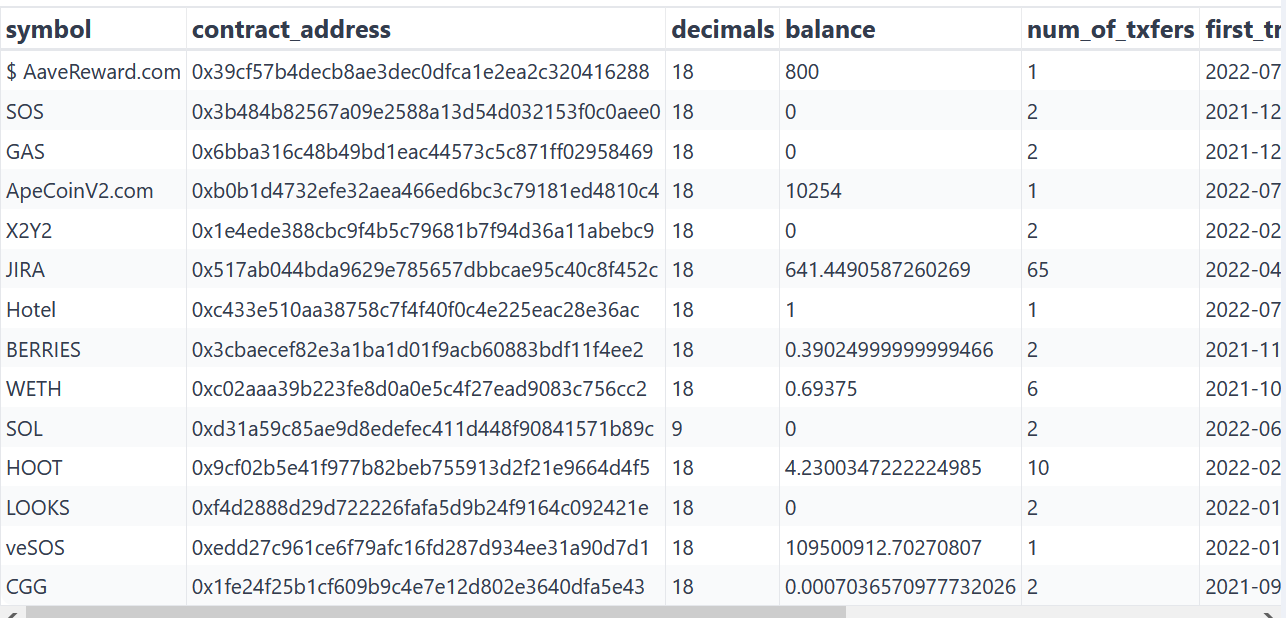## Get NFT Balances for an account

Examples for account `0xdc93be754755d957aa0f5a952504bce24152ccb9`

### Get all NFT balances

``````WITH txfers AS (
SELECT
block_number,
transaction_hash,
symbol,
CASE WHEN from_address = '0xdc93be754755d957aa0f5a952504bce24152ccb9' THEN -1
ELSE 1
END as value,
END as peer,
row_number() OVER(PARTITION BY contract_address ORDER BY block_number DESC) AS row_number,
block_time,
data_creation_date
FROM
ethereum_mainnet.erc721_evt_transfer
WHERE 1=1
AND
-- uncomment to filter on the contract address of the token
ORDER BY block_number DESC
)
SELECT
symbol,
SUM(value) AS balance,
COUNT(1) AS num_of_txfers,
MIN(block_time) AS first_transfer_timestamp,
MAX(block_time) AS last_transfer_timestamp
FROM txfers
-- uncomment to get historical balance at a certain point of time
-- WHERE data_creation_date <= DATE('2022-03-01') AND block_time <= CAST('2022-03-01 06:00' AS timestamp)
LIMIT
200
``````

Output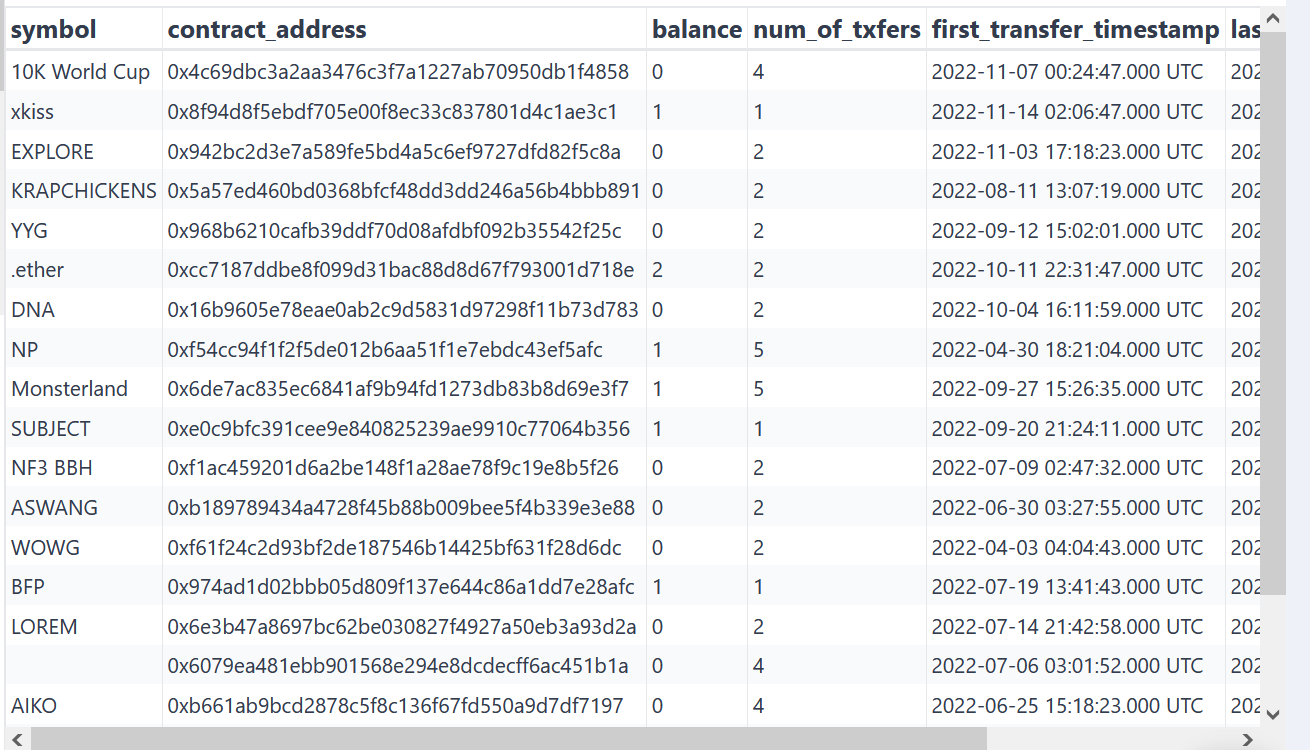### Filter results on NFT token address or symbol

``````...
FROM
ethereum_mainnet.erc721_evt_transfer
WHERE 1=1
AND
ORDER BY block_number DESC
...
``````

### Get historical balances

``````WITH txfers AS (
SELECT
block_number,
transaction_hash,
symbol,
CASE WHEN from_address = '0xdc93be754755d957aa0f5a952504bce24152ccb9' THEN -1
ELSE 1
END as value,
END as peer,
row_number() OVER(PARTITION BY contract_address ORDER BY block_number DESC) AS row_number,
block_time,
data_creation_date
FROM
ethereum_mainnet.erc721_evt_transfer
WHERE 1=1
AND
ORDER BY block_number DESC
)
SELECT
symbol,
SUM(value) AS balance,
COUNT(1) AS num_of_txfers,
MIN(block_time) AS first_transfer_timestamp,
MAX(block_time) AS last_transfer_timestamp
FROM txfers
WHERE data_creation_date <= DATE('2022-03-01') AND block_time <= CAST('2022-03-01 06:00' AS timestamp)
LIMIT
200
``````

Output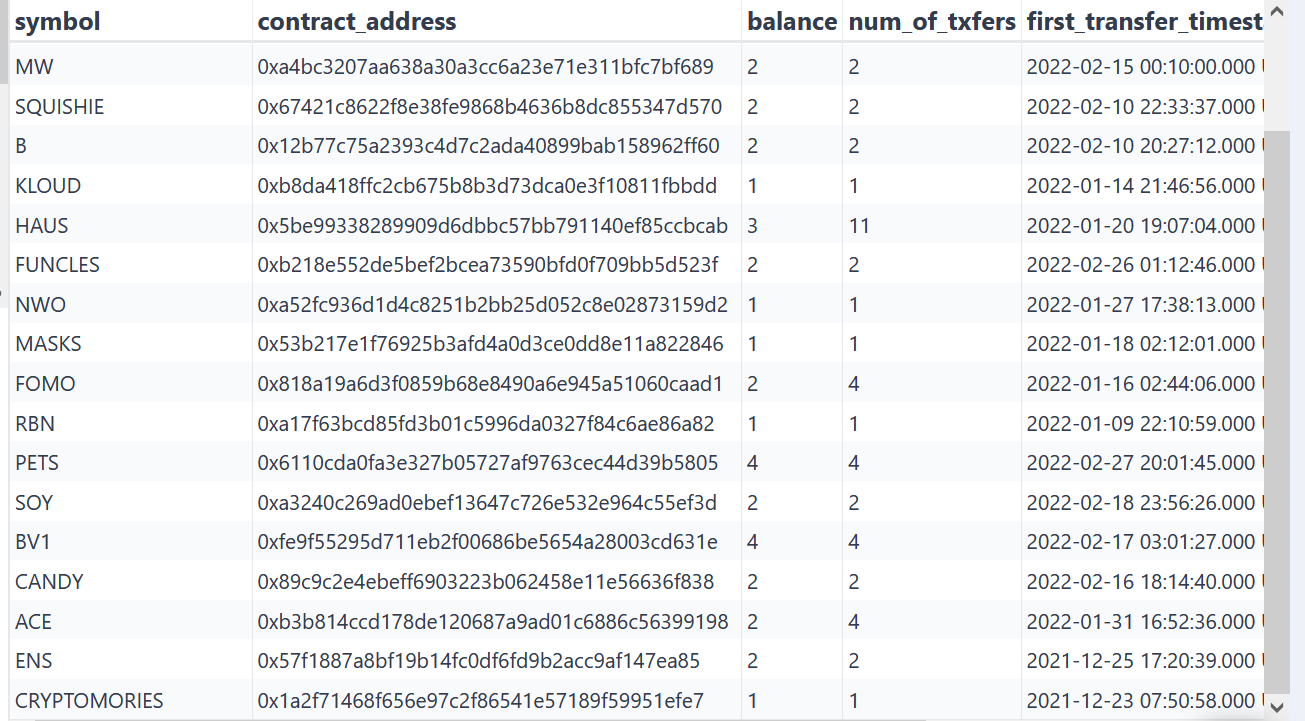## Get All Transactions from a Wallet

Inclusive of price and thereby able to back out the minimum value of a particular wallet in USD or ETH.

The query below pulls all transactions (ERC 20, ERC 1155, and ERC 721) historically for the given wallet address alongside the price.

``````SELECT
'erc20' type,
transaction_hash,
evt_index,
NULL operator,
NULL token_id,
NULL token_id_hex,
VALUE,
symbol,
NULL name,
decimals,
price,
block_number,
block_time,
data_creation_date
FROM
(
SELECT
*
FROM
ethereum_mainnet.erc20_evt_transfer
WHERE
) t
LEFT JOIN (
SELECT
symbol,
DATE(data_creation_date) data_creation_date,
AVG(price) price
FROM
prices.usd
GROUP BY
1,
2
) p USING (symbol, data_creation_date)
UNION
SELECT
'erc721' type,
transaction_hash,
evt_index,
NULL operator,
token_id,
token_id_hex,
'1' VALUE,
symbol,
name,
0 decimals,
NULL price,
block_number,
block_time,
data_creation_date
FROM
ethereum_mainnet.erc721_evt_transfer
WHERE
UNION
SELECT
'erc1155' type,
transaction_hash,
evt_index,
operator,
id token_id,
id_hex token_id_hex,
VALUE,
symbol,
name,
0 decimals,
NULL price,
block_number,
block_time,
data_creation_date
FROM
ethereum_mainnet.erc1155_evt_transfer_single
WHERE
limit 10000
``````

Output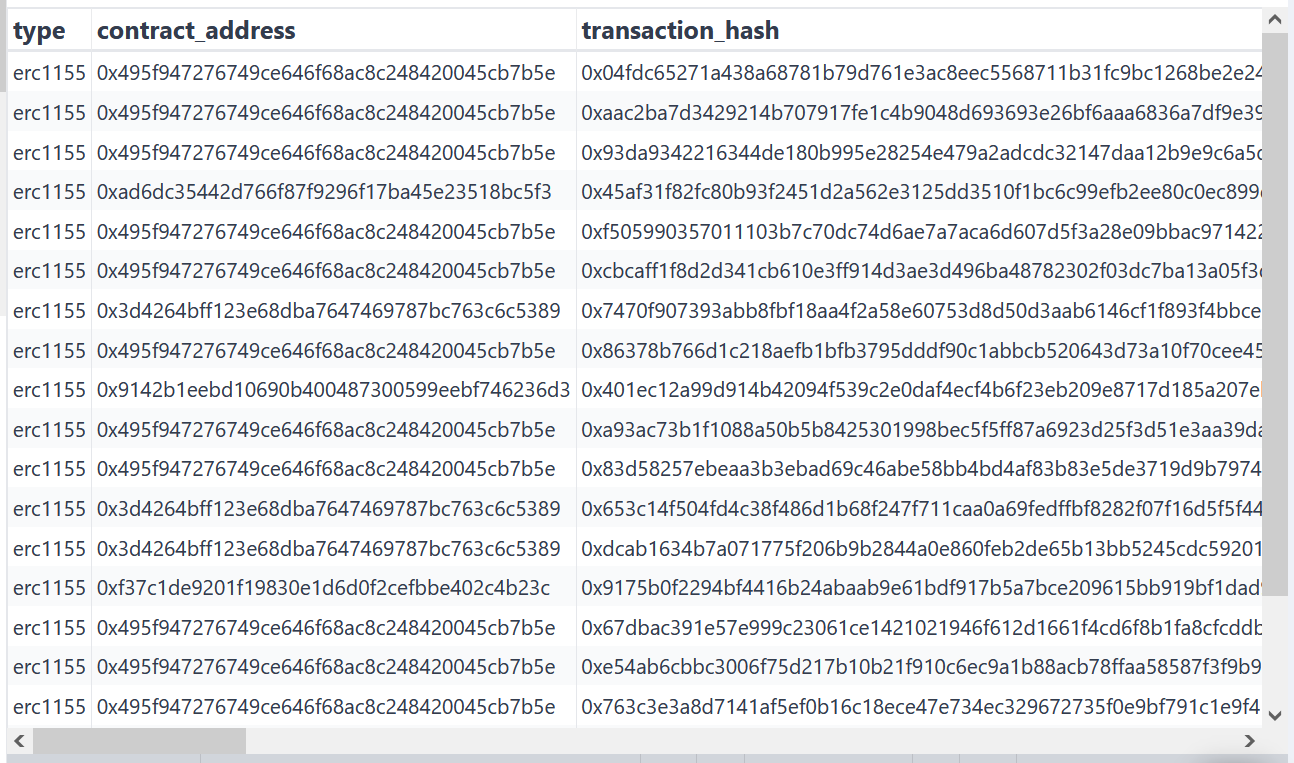## Example: Get USD amount in ERC20 transfers

``````WITH txfers AS (
SELECT
block_number,
transaction_hash,
erc.symbol,
CASE WHEN from_address = '0x3ebd637f0fc8f1bac02cbd138916a8e2d7f5898a' THEN -cast(value as decimal)
ELSE cast(value as decimal)
END as value,
decimals,
END as peer,
price AS usd_price,
row_number() OVER(PARTITION BY contract_address ORDER BY block_number DESC) AS row_number,
block_time,
erc.data_creation_date
FROM
ethereum_mainnet.erc20_evt_transfer erc
LEFT JOIN prices.usd p
ON p.minute = DATE_TRUNC('minute', block_time)
AND lower(p.symbol) = (CASE WHEN lower(erc.symbol) = 'weth' THEN 'eth' ELSE lower(erc.symbol) END)
AND p.data_creation_date = erc.data_creation_date
WHERE 1=1
AND
-- uncomment to filter results on the contract address of the token
ORDER BY block_number DESC
)
SELECT
symbol,
decimals,
SUM(value / pow(10, decimals)) AS balance,
SUM(value / pow(10, decimals) * usd_price) AS usd_balance,
COUNT(1) AS num_of_txfers,
MIN(block_time) AS first_transfer_timestamp,
MAX(block_time) AS last_transfer_timestamp
FROM txfers
-- uncomment to get historical balance at a certain point of time
-- WHERE data_creation_date <= DATE('2022-09-01') AND block_time <= CAST('2022-09-01 06:00' AS timestamp)
LIMIT
200

``````

Output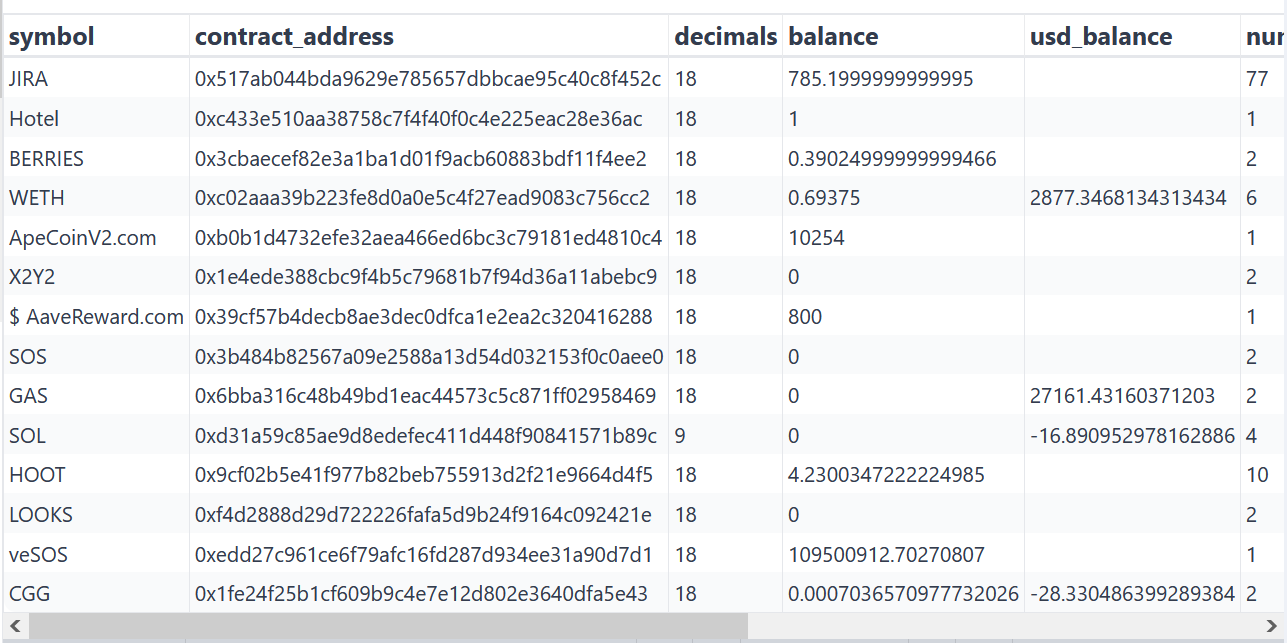## Example: Parse decoded logs

``````SELECT
, transaction_hash as evt_tx_hash
, log_index as evt_index
, block_time as evt_block_time
, block_number as evt_block_number
, argument_values as orderHash
, argument_values as offerer
, argument_values as zone
, argument_values as recipient
, argument_values as offer
, argument_values as consideration
data_creation_date
FROM ethereum_mainnet.logs